# Configuring Output Clocks - 6.0 English

## Clocking Wizard LogiCORE IP Product Guide (PG065)

Document ID
PG065
Release Date
2022-04-20
Version
6.0 English

To enable an output clock, click on the box located next to it. Output clocks must be enabled sequentially. You can rename the output clocks in the output clock table itself.

You can specify values for the output clock frequency, phase shift, and duty cycle assuming that the primary input clock is the active input clock. The Clocking Wizard attempts to derive a clocking network that meets your criteria exactly. In the event that a solution cannot be found, best attempt values are provided and are shown in the actual value column. Actual frequencies are calculated to limit the values to three decimal places. Achieving the specified output frequency takes precedence over implementing the specified phase, and phase in turn takes higher precedence in the clock network derivation process than duty cycle. The precedence of deriving the circuits for the clk_out signals is clk_out1 > clk_out2 > clk_out3 , and so on. Therefore, finding a solution for clk_out1 frequency has a higher priority. Values are recalculated every time an input changes. Because of this, it is best to enter the requirements from top to bottom and left to right. This helps to pinpoint requested values that cannot be supported exactly. If Phase Alignment is selected, the phase shift is with respect to the active input clock.

If a 180° phase shift is requested on clk_out2 , clk_out3 , clk_out4 , or clk_out5 , the Wizard connects any of these clocks to previous clocks. Inverted clock outputs, ( clkout[0:3]B ) of MMCM/PLL, as compared to the previous clock and other properties like frequency, duty cycle, and so on, are identical to the previous clock. If clk_out1 is configured with 100 MHz and a 0° phase shift, and clk_out2 is configured with 100 MHz and 180° phase shift, clk_out2 is connected to clkout0b . If clk_out1 and clk_out2 are 180° phase shifted, and clk_out2 and clk_out3 are 180° phase shifted, clk_out3 uses its own phase settings and is connected to clkout2 of the MMCM. If you have another clock, clk_out4 , with a 180° phase shift compared to clk_out3 , clk_out4 is connected to clkout2b .

You can choose which type of buffer is instantiated to drive the output clocks, or No buffer if the buffer is already available in external code. The buffers available depend on your device family. For all outputs that have BUFR as the output driver, the BUFR_DIVIDE attribute is available as a generic parameter in the HDL. You can change the divide value of the BUFR while instantiating the design.

Note: The Max Freq. of buffer column in the Output Clocks tab of the Clocking Wizard (as shown in This Figure ) shows the maximum frequency of the clock that the selected output buffer can drive. When you select a buffer, ensure that the required frequency is within the range of frequencies that the buffer can support. Otherwise, you might get timing violations.

If you select Dynamic Phase Shift clocking, the Use Fine PS check boxes become available. 'These checkboxes allow you to enable the variable fine phase shift on the MMCM(E2/E3). Select the appropriate check box for any clock that requires dynamic phase shift. The Wizard resets the requested phase field to 0.000 when Use Fine PS is selected.

When the Safe Clock Startup feature is enabled on the first tab of the GUI, the Use Clock Sequencing table is active and the sequence number for each enabled clock is available for configuration. In this mode, only BUFGCE is allowed as a driver of the clock outputs.

Both 7 series and UltraScale devices support the MMCM fractional divide functionality in increments of 1/8th (0.125) for CLKFBOUT and CLKOUT0 , and can support greater clock frequency synthesis. The resolution of the fractional divide is 1/8 or 0.125 degrees, effectively increasing the number of synthesizable frequencies by a factor of eight. For example, if the CLKIN frequency is 100 MHz and the M divide value is set to 8, the VCO frequency is 800 MHz. CLKOUT0 can be used to further fractionally divide the 800 MHz VCO frequency (for example, CLKOUT0_DIVIDE = 2.5, resulting in a 320 MHz output frequency).

Note: The fractional divide values entered in override mode must be in multiples of 0.125. Otherwise, the IP returns an error saying that the value must be a multiple of 0.125.

When using the fractional divider, the duty cycle is not programmable for outputs used in the fractional mode. Fractional divide is not allowed in fixed or dynamic phase shift mode. The CDDC feature is not available in the fractional divide mode for UltraScale devices. See 7 Series FPGAs Clocking Resources User Guide (UG472) [Ref 9] and UltraScale Architecture Clocking Resources User Guide (UG572) [Ref 8] for more information.

 X-Ref Target - Figure 4-5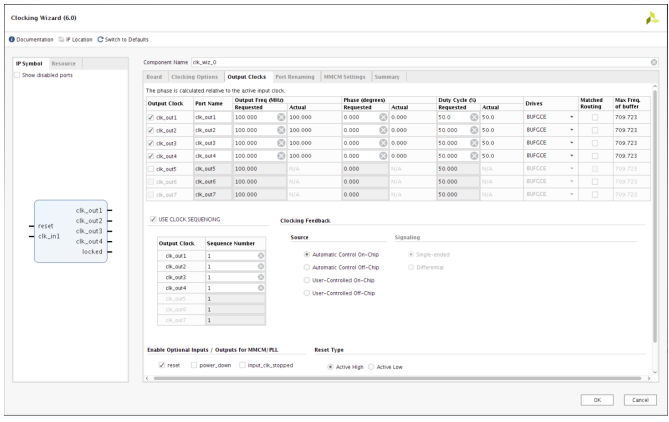You can configure the sequence number from 1 to the maximum number of clocks selected. The Clocking Wizard does not allow any break in the sequence from one to the maximum in the table. The frequency of the output clock in the sequence must not be more than eight times that of the output clock next in sequence. For details of the clocking behavior in this mode, see This Figure and This Figure .

 X-Ref Target - Figure 4-6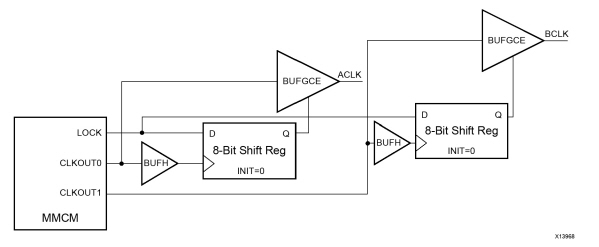X-Ref Target - Figure 4-7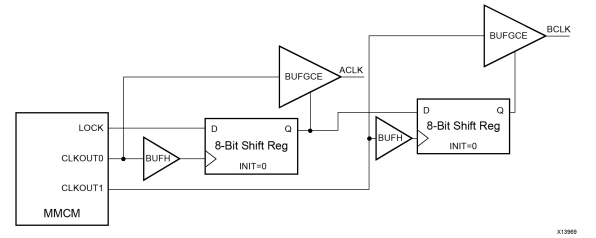When Spread Spectrum (SS) is selected, CLK_OUT<3> and CLK_OUT<4> are not available. Divide values of these outputs are used for SS modulation frequency generation.

 X-Ref Target - Figure 4-8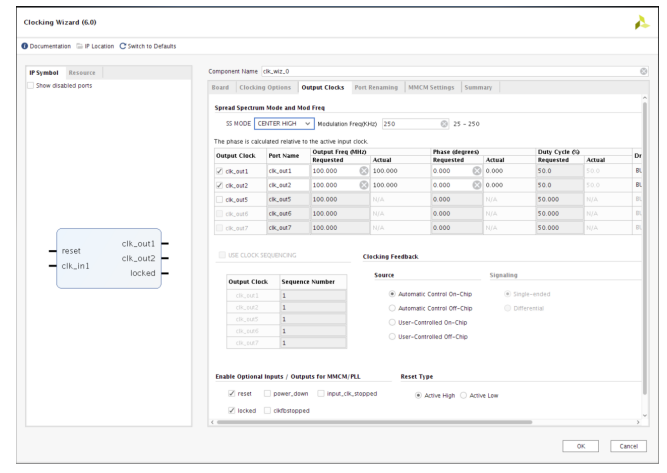There are four modes available for SS mode:

DOWN_LOW

DOWN_HIGH

CENTER_LOW

CENTER_HIGH

The available modulation frequency range is 25 – 250 KHz. Spread spectrum calculation details are described in This Figure and This Figure .

 X-Ref Target - Figure 4-9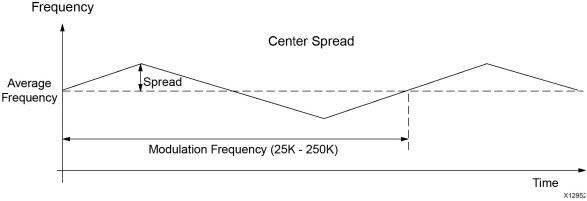X-Ref Target - Figure 4-10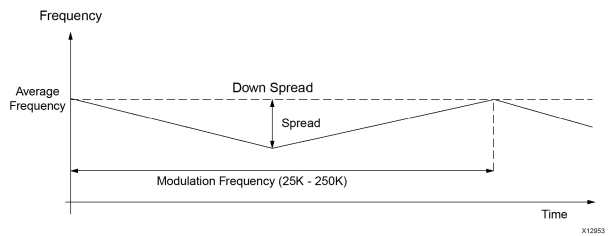Note: Input_clock_frequency is in Hz.

If (SS_Mode = CENTER_HIGH) :=>

° spread (ps) = +/- [1/(Input_clock_frequency*(M-0.125*4)/D/O) - 1/(Input_clock_frequency*M/D/O)]

If (SS_Mode = CENTER_LOW) :=>

° spread (ps) = +/- [1/(Input_clock_frequency*(M-0.125*4)/D/O) - 1/(Input_clock_frequency*M/D/O)]

If (SS_Mode = DOWN_HIGH) :=>

° spread (ps) = + [1/(Input_clock_frequency*(M-0.125*4)/D/O) - 1/(Input_clock_frequency*M/D/O)]

If (SS_Mode = DOWN_LOW) :=>

° spread (ps) = + [1/(Input_clock_frequency*(M-0.125*4)/D/O) - 1/(Input_clock_frequency*M/D/O)]

Where M is CLKFBOUT_MULT_F, D is DIVCLK_DIVIDE, and O is respective CLKOUTx_DIVIDE.

For modulation frequency:

° O2 and O3 are calculated by the BitGen in implementation. The same calculation is done in the Wizard to get an actual modulation frequency value.

° Based on how O2 and O3 are calculated, the actual modulation frequency is calculated:

If (SS_Mode = CENTER_HIGH or SS_Mode = CENTER_LOW) Actual_modulation_frequency (average) = (Input_clock_frequency*M/D) / (O2 * O3) / 16

If (SS_Mode = DOWN_HIGH) Actual_modulation_frequency (average) = 0.5 * [((Input_clock_frequency*M/D) / (O2 * O3) / 8) + ((Input_clock_frequency*(M-0.5)/D) / (O2 * O3) / 8)]

If (SS_Mode = DOWN_LOW) Actual_modulation_frequency (average) = 0.5 * [((Input_clock_frequency*M/D) / (O2 * O3) / 8) + ((Input_clock_frequency*(M-0.25)/D) / (O2 * O3) / 8)]

IMPORTANT: The actual modulation frequency might deviate within +/- 10% of the requested modulation frequency for some settings.